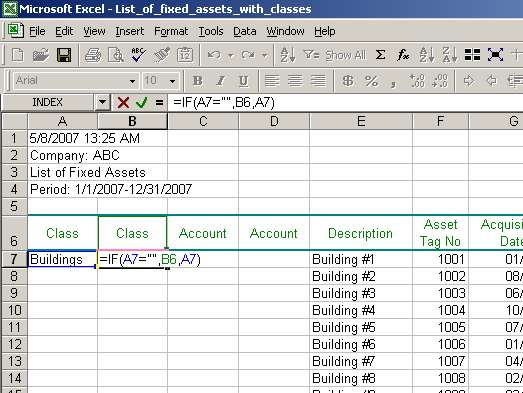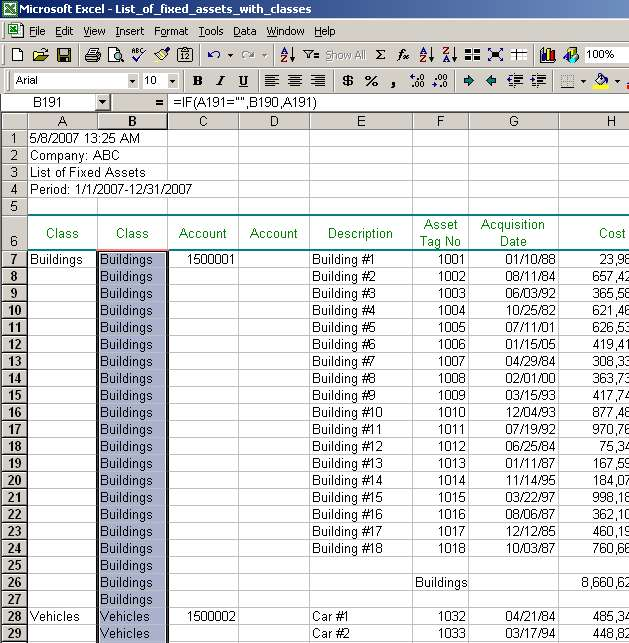# Excel Fill Down Function, Excel Fill Down Examples

### 4) Use Excel formula IF to fill down empty cells

We are ready to fill in the empty cells. As was mentioned before, one could have copied and pasted values in columns Class and Account manually for each class and account. However, if there are too many of them, this task may take a lot of time and significantly decrease our productivity. Instead, we can use a formula to do the job for us. Let us look at the below formula which we first will enter in cell B7 (see tab Step 4 in our listing of fixed assets):

=IF(A7="",B6,A7)The logic of the formula above is as follows. If cell A7 equals an empty string (=""), then the formula assigns value of cell B6 to cell B7; otherwise (if cell A7 is not empty), it assigns value of cell A7 to cell B7. It does not seem like the formula does much. However, let us copy and paste this formula in column B until end of data (row 191) and see what happens:Page 4 of 6